# Brilliant Junior Integration Contest - Season 1

Hi Brilliant! Just like what Aditya Kumar and Anastasiya Romanova conducted earlier, this year we would also like to conduct an integration contest for juniors.

The aims of the Integration contest are to improve skills in the computation of integrals, to learn from each other as much as possible, and of course to have fun.

Eligibility:- People should fulfill either of the 2 following

• 17 years or below

• Level 4 or below in Calculus

Eligible people here may participate in this contest.

The rules are as follows:

• I will start by posting the first problem. If there is a user solves it, then they must post a new one.

• You may only post a solution of the problem below the thread of problem and post your proposed problem in a new thread. Put them separately.

• Only make substantial comment that will contribute to the discussion.

• Make sure you know how to solve your own problem before posting it in case there is no one can answer it within 48 hours, then you must post the solution and you have a right to post another problem.

• If the one who solves the last problem does not post his/her own problem after solving it within a day, then the one who has a right to post a problem is the last solver before him/her.

• The scope of questions is only computation of integrals either definite or indefinite integrals.

• It is NOT compulsory to post original problems. But make sure it has not been posted on brilliant.

• Do not copy questions from last year's contest. If anyone found to do so he/she will be banned from taking further part in this contest

• You are also NOT allowed to post a solution using a contour integration or residue method.

The final answer can ONLY contain the following special functions: gamma function, beta function, Riemann zeta function, digamma function,Harmonic numbers, trigonometric integral, Wallis' integral,

Format your post is as follows:

 1 2 3 4 5 6 7 **SOLUTION OF PROBLEM xxx (number of problem) :** **[Post your solution here]** **PROBLEM xxx (number of problem) :** **[Post your problem here]** 

The comments will be easiest to follow if you sort by "Newest":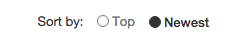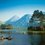Note by Rajdeep Dhingra
5 years, 4 months ago

This discussion board is a place to discuss our Daily Challenges and the math and science related to those challenges. Explanations are more than just a solution — they should explain the steps and thinking strategies that you used to obtain the solution. Comments should further the discussion of math and science.

When posting on Brilliant:

• Use the emojis to react to an explanation, whether you're congratulating a job well done , or just really confused .
• Ask specific questions about the challenge or the steps in somebody's explanation. Well-posed questions can add a lot to the discussion, but posting "I don't understand!" doesn't help anyone.
• Try to contribute something new to the discussion, whether it is an extension, generalization or other idea related to the challenge.

MarkdownAppears as
*italics* or _italics_ italics
**bold** or __bold__ bold
- bulleted- list
• bulleted
• list
1. numbered2. list
1. numbered
2. list
Note: you must add a full line of space before and after lists for them to show up correctly
paragraph 1paragraph 2

paragraph 1

paragraph 2

[example link](https://brilliant.org)example link
> This is a quote
This is a quote
    # I indented these lines
# 4 spaces, and now they show
# up as a code block.

print "hello world"
# I indented these lines
# 4 spaces, and now they show
# up as a code block.

print "hello world"
MathAppears as
Remember to wrap math in $$ ... $$ or $ ... $ to ensure proper formatting.
2 \times 3 $2 \times 3$
2^{34} $2^{34}$
a_{i-1} $a_{i-1}$
\frac{2}{3} $\frac{2}{3}$
\sqrt{2} $\sqrt{2}$
\sum_{i=1}^3 $\sum_{i=1}^3$
\sin \theta $\sin \theta$
\boxed{123} $\boxed{123}$

Sort by:

Problem 18:

Prove that: $\int_{0}^{1} \left(\frac{1}{\log x} + \frac{1}{1-x} -\frac{1}{2} \right) \frac{ \mathrm{d}x}{1-x} = -\frac{1}{2}+\frac{1}{2} \ln (2 \pi)-\frac{1}{2} \gamma.$

The Problem has been solved by Ishan Singh

- 5 years, 4 months ago

Lemma 1 : $\displaystyle \sum_{r=1}^{n} H_{r} = (n+1)H_{n} - n$

Proof : $\displaystyle \sum_{r=1}^{n} H_{r} = \int_{0}^{1} \sum_{r=1}^{n} \dfrac{x^r-1}{x-1} \mathrm{d}x$

$\displaystyle = \int_{0}^{1} \dfrac{x^{n+1} - x - nx + n}{(x-1)^2} \mathrm{d}x$

$\displaystyle = \int_{0}^{1} \dfrac{x^{n+1} - x}{(x-1)^2} \mathrm{d}x - \int_{0}^{1} \dfrac{n}{x-1} \mathrm{d}x$

$\displaystyle = \text{A} + \text{B}$

Using Integration By Parts on the first integral $\text{A}$, we have,

$\displaystyle \text{A} = -\left[\dfrac{x^{n+1}-x}{x-1}\right]_{0}^{1} + \int_{0}^{1}\dfrac{(n+1)x^n - 1}{x-1} \mathrm{d}x$

$\displaystyle = -n + \int_{0}^{1}\dfrac{(n+1)x^n - 1}{x-1} \mathrm{d}x$

$\displaystyle \implies \text{A} +\text{B} = -n + (n+1) \cdot \int_{0}^{1}\dfrac{x^n - 1}{x-1} \mathrm{d}x$

$\displaystyle = (n+1)H_{n} - n$

Lemma 2 : $\displaystyle \int_{0}^{1} \dfrac{x^n - 1}{\ln x} \mathrm{d}x = \ln (n +1)$

Proof : $\displaystyle \int_{0}^{1} \dfrac{x^n - 1}{\ln x} \ \mathrm{d}x = \int_{0}^{1} \int_{0}^{n} x^y \ \mathrm{d}y \ \mathrm{d}x$

$\displaystyle = \int_{0}^{n} \int_{0}^{1} x^y \ \mathrm{d}x \ \mathrm{d}y$

$\displaystyle = \int_{0}^{n} \dfrac{1}{y+1} \ \mathrm{d}y$

$\displaystyle = \ln (n+1)$

Lemma 3 : $\displaystyle \int_{0}^{1} \left(\dfrac{1}{\ln (x)} + \dfrac{1}{1-x}\right) \mathrm{d}x = \gamma$

Proof : $\displaystyle \int_{0}^{1} \left( \dfrac{1}{1-x} + \dfrac{1}{\ln (x)}\right) \mathrm{d}x = \lim_{n \to \infty} \int_0^1\left(\dfrac{1-t^n}{1-t}-\frac{t^{n-1}-1}{\ln t} \mathrm{d}t \right)$

$\displaystyle = \lim _{n \to \infty} (H_{n} - \ln n)$

$\displaystyle = \gamma$

Now,

$\displaystyle \text{I} = \int_{0}^{1} \left(\dfrac{1}{\ln x} + \dfrac{1}{1-x} - \dfrac{1}{2}\right)\dfrac{\mathrm{d}x}{x-1}$

$\displaystyle = \lim_{n \to \infty} \sum_{r=1}^{n} \int_{0}^{1} \left(\dfrac{x^{r-1}}{\ln x} + \dfrac{x^{r-1}}{1-x} - \dfrac{x^{r-1}}{2} \right)$

Using Lemma 2 and Lemma 3 , we have,

$\displaystyle \text{I} = \lim_{n \to \infty} \sum_{r=1}^{n} \left(\gamma + \ln r - H_{r-1} -\dfrac{1}{2r} \right)$

$\displaystyle = \lim_{n \to \infty} \left( n\gamma + \ln (n!) - \sum_{r=1}^{n} H_{r-1} - \dfrac{H_{n}}{2} \right)$

Using Lemma 1,

$\displaystyle \text{I} = \lim_{n \to \infty} \left( n\gamma + \ln (n!) -n H_{n-1} + n - 1 -\dfrac{H_{n}}{2} \right)$

Using Stirling's Approximation, we have,

$\displaystyle \text{I} = \lim_{n \to \infty} \left( n\gamma + \dfrac{1}{2} \ln (2\pi) - n +\dfrac{1}{2} \ln (n) + n\ln (n) -n H_{n-1} + n - 1 -\dfrac{H_{n}}{2} \right)$

Simplifying using $\displaystyle \lim_{n \to \infty} (H_{n} - \ln n) = \gamma$, we have,

$\displaystyle \text{I} = \boxed{\dfrac{1}{2} \ln (2\pi) -\dfrac{1}{2} \gamma - \dfrac{1}{2}}$

- 5 years, 4 months ago

wow,that was probably why i gave up halfway through lol

i'm sorry i didn't attend the integration contest until now,there was a problem with my wifi and it got fixed

- 5 years, 4 months ago

- 5 years, 4 months ago

@Aditya Kumar May post another question if he wants.

- 5 years, 4 months ago

Nice. I used Binet's formula.

- 5 years, 4 months ago

Problem 1

Find the value of the following $\Large \displaystyle \dfrac\int_{-\infty}^{\infty}{{(x+1)}^2 e^{-{(x+1)}^2}dx}}\int_{-\infty}^{\infty}{e^{-x^2}dx}$

This problem has been solved by Aditya Kumar.

- 5 years, 4 months ago

Solution to Problem 1: ${ I }_{ 1 }=\int _{ -\infty }^{ \infty }{ { \left( x+1 \right) }^{ 2 }{ e }^{ { -\left( x+1 \right) }^{ 2 } } } dx$

Substitute: ${ \left( x+1 \right) }^{ 2 }=t$

Therefore, we get: ${ I }_{ 1 }=\frac { 1 }{ 2 }\int _{ -\infty }^{ \infty }{ { \left( t \right) }^{ \frac { 1 }{ 2 } }{ e }^{ { - }t } } dt$

This is symmetric about $x=0$. Therefore, we can write it as: ${ I }_{ 1 }=\int _{ 0 }^{ \infty }{ { \left( t \right) }^{ \frac { 1 }{ 2 } }{ e }^{ { - }t } } dt$

${ I }_{ 1 }=\Gamma \left( \frac { 3 }{ 2 } \right) =\frac { 1 }{ 2 } \Gamma \left( \frac { 1 }{ 2 } \right) =\frac { \sqrt { \pi } }{ 2 }$

Similarly, ${ I }_{ 2 }=\sqrt { \pi }$

Hence, $\boxed{\frac{{ I }_{ 1 }}{{ I }_{ 2 }}=\frac{1}{2}}$

- 5 years, 4 months ago

Problem 2:

Prove that: $\int _{ 0 }^{ 1 }{ \frac { \arctan { \left( \sqrt { { x }^{ 2 }+2 } \right) } }{ \sqrt { { x }^{ 2 }+2 } \left( { x }^{ 2 }+1 \right) } \ dx } =\frac { 5{ \pi }^{ 2 } }{ 96 }$

This problem has been solved by Harsh Shrivastava.

- 5 years, 4 months ago

Solution to Problem 2

$I = \int _{ 0 }^{ 1 }{ \frac { \arctan { \left( \sqrt { { x }^{ 2 }+2 } \right) } }{ \sqrt { { x }^{ 2 }+2 } \left( { x }^{ 2 }+1 \right) } \ dx } =\frac { 5{ \pi }^{ 2 } }{ 96 }$

Lets split $I$ as $A \text{ and } B$ by using the property that $\arctan(x) = \pi /2 -\arctan(1/x)$

Thus our integral becomes $I = A-B$,

where $A = \displaystyle \pi /2\int_{0}^{1}\dfrac{dx}{(1+x^{2})(\sqrt{2+x^{2}})}$

and $B= \displaystyle \int_{0}^{1}\dfrac{\arctan(\frac{1}{\sqrt{x^{2} + 2}})}{(1+x^{2})(\sqrt{2+x^{2}})}$

Evaluating A,

Let's evaluate A, without the limits,

$\displaystyle \pi /2\int\dfrac{dx}{(1+x^{2})(\sqrt{2+x^{2}})} = \pi /2\arctan \dfrac{x}{\sqrt{x^{2} + 2}}$

Which on substituting limits gives A = $\pi ^{2}/12$

Evaluating B,

Using $1/a \arctan(1/a) = \int_{0}^{a} \frac{dy}{y^{2}+a^{2}}$,

Let $a = \sqrt{x^2+2}$.

We get $B = \displaystyle \int_{0}^{1}\int_{0}^{1} \dfrac{dxdy}{(1+x^{2})(2+x^{2} + y^{2})}$

$B = \displaystyle \int_{0}^{1}\int_{0}^{1} \dfrac{dxdy}{(1+x^{2})(1+y^{2})} - \int_{0}^{1}\int_{0}^{1} \dfrac{dxdy}{(1+x^{2})(2+x^{2} + y^{2})}$

$2B = \displaystyle \int_{0}^{1}\int_{0}^{1} \dfrac{dxdy}{(1+x^{2})(1+y^{2})}$

$2B = \displaystyle (\int_{0}^{1}\dfrac{dx}{1+x^{2}})^{2}$

Thus $B = \pi^{2} / 32$

Hence $I = A-B=\dfrac{5\pi^{2}}{96}$

- 5 years, 4 months ago

- 5 years, 4 months ago

Problem 14:

If $\displaystyle \int_{0}^{\infty} \dfrac{x^{3}}{1+e^{\alpha x}}dx = \dfrac{1}{64}$
Find $\alpha$ given that , $\alpha$ is a positive constant and $\zeta(4) = \dfrac{\pi^{4}}{90}$

Problem has been solved by Rajdeep Dhingra and Aareyan Manzoor at the same instant. Aareyan has been given credits due to his solution.

- 5 years, 4 months ago

$I=\int_0^\infty x^3 \sum_{n=1}^\infty (-e^{-\alpha x})^n dx$ $I= \sum_{n=1}^\infty (-1)^n \int_0^\infty x^3 e^{-\alpha n x}dx=\sum_{n=1}^\infty (-1)^n \dfrac{6}{(\alpha n)^4}$ $I=\dfrac{6}{\alpha^4} (1-2^{-3}) \zeta(4)=\dfrac{1}{64}$ the result follows.

- 5 years, 4 months ago

Is the answer $\huge \displaystyle \alpha = \pi {\left(\frac{56}{15}\right)}^{\frac14}$ ?

- 5 years, 4 months ago

Problem 6:

Find a closed form of the indefinite integral $\int H_{x} dx$

Where $H_x$ denotes harmonic number

This problem has been solved by Aditya Kumar.

- 5 years, 4 months ago

Solution to Problem 6:

I'll use the definition: ${ H }_{ x }=\psi \left( x+1 \right) +\gamma$

$\int { { H }_{ x }dx } =\int { \left( \psi \left( x+1 \right) +\gamma \right) dx }$

We know that $\displaystyle \psi \left( x \right) =\frac { d\left( x! \right) }{ dx }$. Hence, using this, we get: $\boxed\int { { H }_{ x }dx } =ln\left( x! \right) +\gamma x+c$

- 5 years, 4 months ago

Problem 10

Let $S = \sin^2{(x)} + \dfrac{sin^4{(x)}}{3^2} + \dfrac{sin^6{(x)}}{5^2} + \cdots \text{ Upto Infinity }$

Prove that $\int_{0}^{\pi /2} {S \ dx} = \dfrac{{\pi}^2}{4} - \dfrac{\pi}2$

The Problem has been solved by Ishan Singh.

- 5 years, 4 months ago

I have come up with several ways to prove it. Here is one of the more interesting methods.

Lemma: $\displaystyle f(a) = \dfrac{1}{1+a} + \sum_{r=1}^{\infty} \left[ \dfrac{1}{(2r+a+1)}\prod_{k=1}^{r}\left(\dfrac{2k-1}{2k}\right) \right] = \dfrac{\pi}{2^{a+1}} \dfrac{\Gamma (a+1)}{\Gamma^2\left(\dfrac{a+2}{2}\right)} \quad ; \quad a > -1$

Proof: I have proved it here

Now,

$\displaystyle \text{I} = \int_{0}^{\pi /2} {S \ \mathrm{d}x} = \sum_{r=1}^{\infty}\int_{0}^1 \dfrac{\sin ^{2r}}{(2r-1)^2} \mathrm{d}x$

$\displaystyle = \dfrac{1}{2} \sum_{r=1}^{\infty} \dfrac{\operatorname{B}\left(r+\frac{1}{2} , \frac{1}{2} \right)}{(2r-1)^2}$

$\displaystyle = \dfrac{1}{2} \sum_{r=1}^{\infty} \dfrac{\Gamma\left(r+\frac{1}{2} \right) \Gamma \left(\frac{1}{2} \right)}{(2r-1)^2 \Gamma(r+1)}$

$\displaystyle = \dfrac{\pi}{2} \sum_{r=1}^{\infty} \dfrac{\Gamma\left(r+\frac{1}{2} \right) }{(2r-1)^2 \Gamma \left(\frac{1}{2}\right) \Gamma(r+1)}$

Changing the index of summation and using $\Gamma(x+1) = x\Gamma(x)$, we have,

$\displaystyle \text{I} = \dfrac{\pi}{4} \sum_{r=0}^{\infty} \dfrac{\Gamma\left(r+\frac{1}{2} \right)}{(r+1)(2r+1) \Gamma \left(\frac{1}{2}\right) \Gamma(r+1)}$

$\displaystyle = \dfrac{\pi}{2} \left[\sum_{r=0}^{\infty} \dfrac{\Gamma\left(r+\frac{1}{2} \right)}{(2r+1) \Gamma \left(\frac{1}{2}\right) \Gamma(r+1)} - \sum_{r=0}^{\infty} \dfrac{\Gamma\left(r+\frac{1}{2} \right)}{(2r+2) \Gamma \left(\frac{1}{2}\right) \Gamma(r+1)}\right]$

Using my solution here, we have,

$\displaystyle \text{I} = \dfrac{\pi}{2} \left[ 1+ \sum_{r=1}^{\infty} \dfrac{1}{(2r+1)} \prod_{k=1}^{r}\left(\dfrac{2k-1}{2k}\right) - \dfrac{1}{2} - \sum_{r=1}^{\infty} \dfrac{1}{(2r+2)} \prod_{k=1}^{r}\left(\dfrac{2k-1}{2k}\right) \right]$

$\displaystyle = \dfrac{\pi}{2} \left[f(0) - f(1) \right]$

$\displaystyle = \boxed{\dfrac{\pi ^2}{4} - \dfrac{\pi}{2}}$

- 5 years, 4 months ago

- 5 years, 4 months ago

More or less the same.

- 5 years, 4 months ago

@Ishan Singh @Rajdeep Dhingra Do any of you know another method to solve this question?

- 5 years, 4 months ago

Ishan wrote that he has come up with several ways so I guess has many. @Ishan Singh

- 5 years, 4 months ago

My other methods include using Dilogarithm and derivative of Beta function.

- 5 years, 4 months ago

Note to the Participants:

• Please refrain from posting solution through images. It makes this note slow to load.

• Up-vote good solutions and problems. I have see that people are not up-voting at all. This is a wrong sign in the contest. Up-voting encourages the users to improve.

- 5 years, 4 months ago

Very True.I will change the note after it crosses 50 questions.

- 5 years, 4 months ago

Maybe you should do it now, my browser has hanged twice loading this note!

- 5 years, 4 months ago

Change it after 20. 50 is too much.

- 5 years, 4 months ago

Problem 17

Prove that if $c < 1$, $\int_{0}^{\pi/2} {\arcsin{(c \cos{(x)})} \ dx} = \frac{c}{1^2} + \frac{c^3}{3^2} + \frac{c^5}{5^2} + \cdots \text{ (Upto Infinity)}$

The Problem has been solved by Aditya Kumar

- 5 years, 4 months ago

Solution to Problem 17:

Maclaurin series of $\arcsin { \left( x \right) }$ is: $\displaystyle \arcsin { \left( x \right) } =\sum _{ n=0 }^{ \infty }{ \frac { \Gamma \left( n+\frac { 1 }{ 2 } \right) }{ \sqrt { \pi } \left( 2n+1 \right) n! } { x }^{ 2n+1 } }$

Here $x=c \cos(x)$

$\int _{ 0 }^{ \frac { \pi }{ 2 } }{ \arcsin { \left( c\cos { \left( x \right) } \right) } dx } =\int _{ 0 }^{ \frac { \pi }{ 2 } }{ \sum _{ n=0 }^{ \infty }{ \frac { \Gamma \left( n+\frac { 1 }{ 2 } \right) }{ \sqrt { \pi } \left( 2n+1 \right) n! } { \left( c\cos { \left( x \right) } \right) }^{ 2n+1 } } dx }$

$\int _{ 0 }^{ \frac { \pi }{ 2 } }{ \arcsin { \left( c\cos { \left( x \right) } \right) } dx } =\sum _{ n=0 }^{ \infty }{ \frac { \Gamma \left( n+\frac { 1 }{ 2 } \right) { c }^{ 2n+1 } }{ \sqrt { \pi } \left( 2n+1 \right) n! } \int _{ 0 }^{ \frac { \pi }{ 2 } }{ { \left( \cos { \left( x \right) } \right) }^{ 2n+1 }dx } }$

Therefore, by beta function we get:

$\int _{ 0 }^{ \frac { \pi }{ 2 } }{ \arcsin { \left( c\cos { \left( x \right) } \right) } dx } =\sum _{ n=0 }^{ \infty }{ \left( \frac { \Gamma \left( n+\frac { 1 }{ 2 } \right) { c }^{ 2n+1 } }{ \sqrt { \pi } \left( 2n+1 \right) n! }\frac { 1 }{ 2 } \frac { \Gamma \left( \frac { 1 }{ 2 } \right) \Gamma \left( n+1 \right) }{ \Gamma \left( n+\frac { 3 }{ 2 } \right) } \right) }$

Hence, on simplifying, we get: $\boxed{ \displaystyle \int_{0}^{\pi/2} {\arcsin{(c \cos{(x)})} \ dx} = \frac{c}{1^2} + \frac{c^3}{3^2} + \frac{c^5}{5^2} + \cdots \text{ (Upto Infinity)}}$

- 5 years, 4 months ago

Problem 9

Prove $\int_0^{\pi/2} x\ln(\tan(x)) dx= \dfrac{7\zeta(3)}{8}$

The Problem has been solved by Rajdeep Dhingra.

- 5 years, 4 months ago

Solution to Problem 9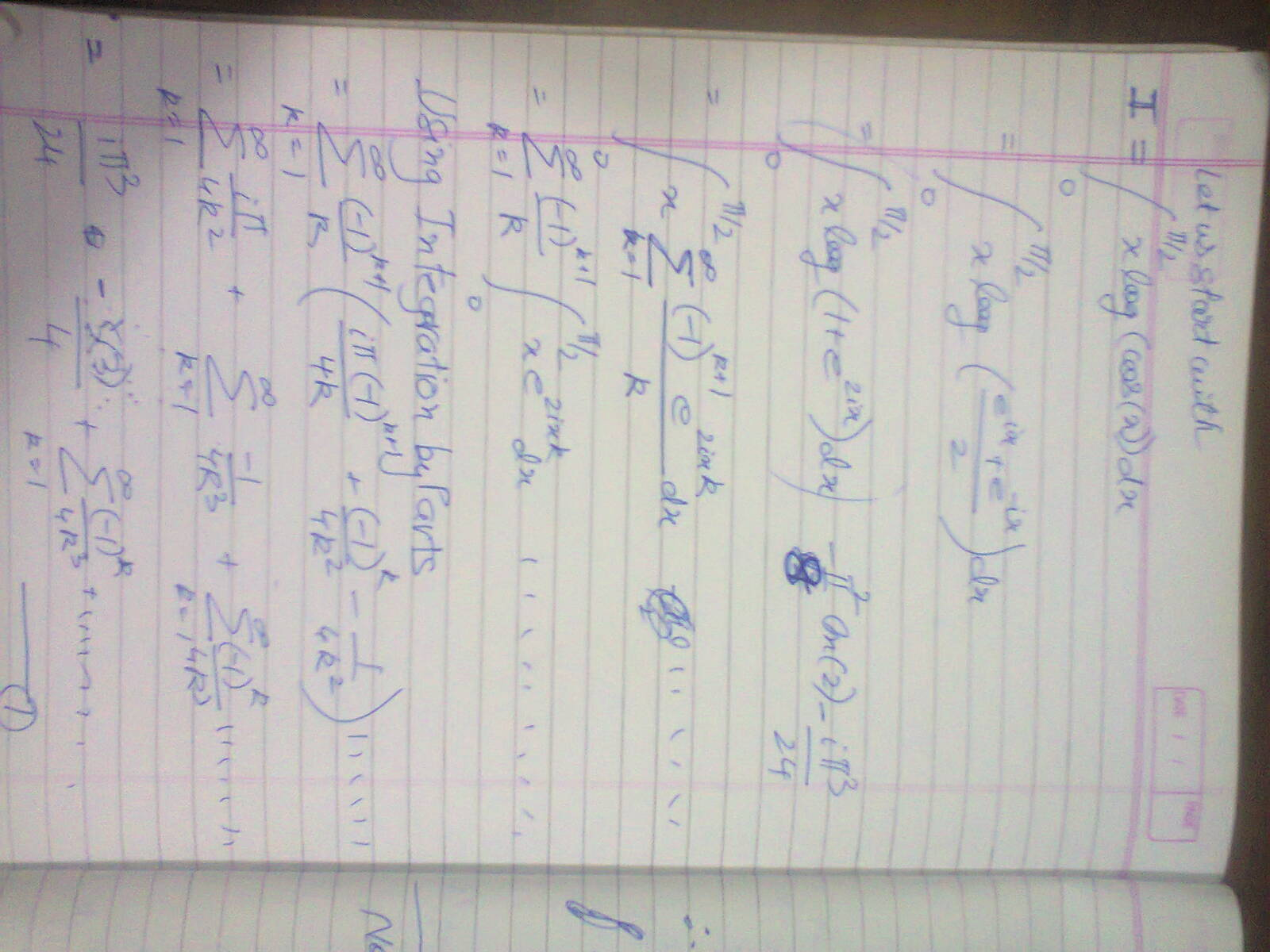Page 1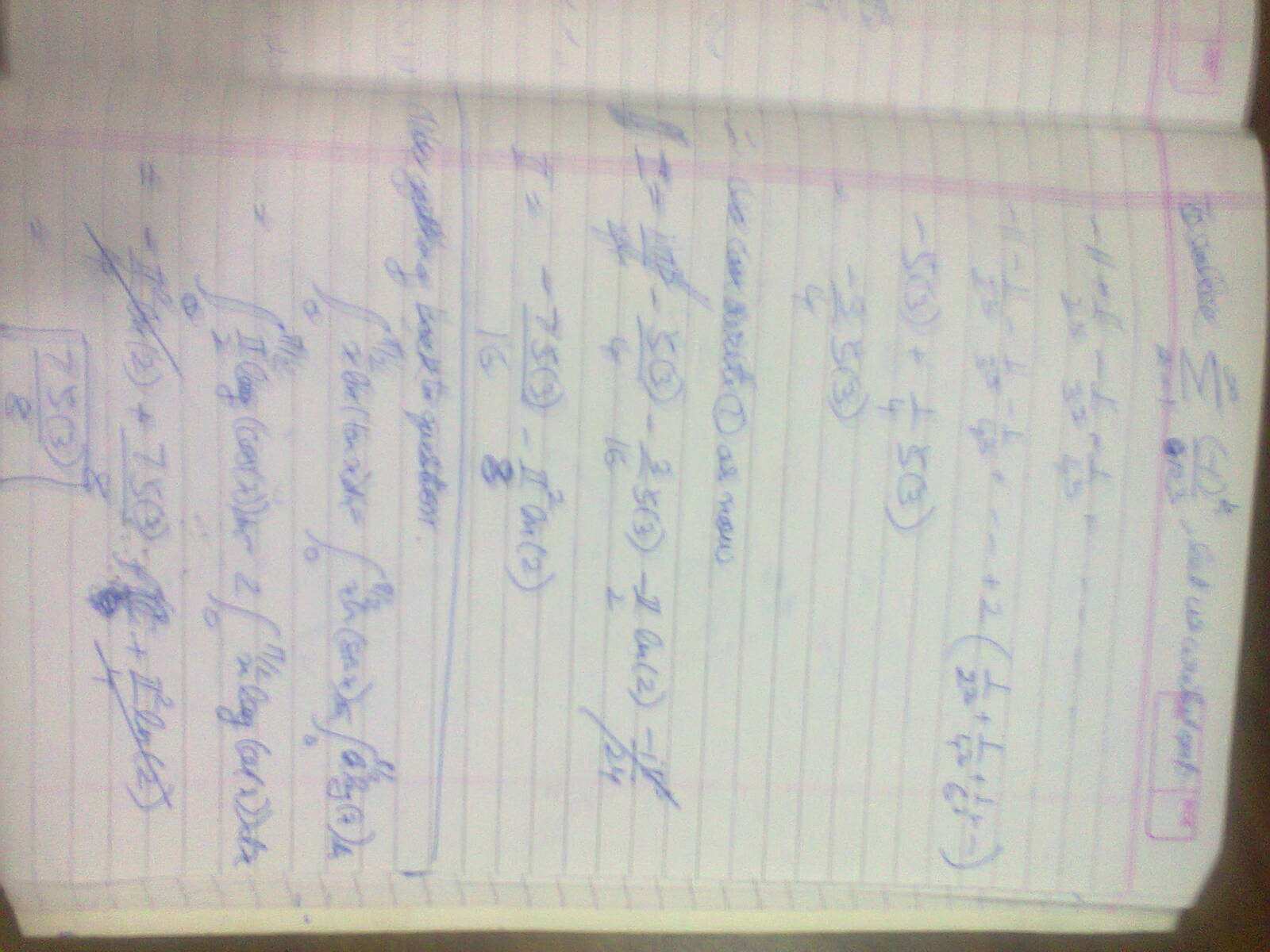Page 2

- 5 years, 4 months ago

The second page is not at all clear. Please LaTeX your solution.

- 5 years, 4 months ago

Problem 23

Prove that for an integer $n\ge2$

$\displaystyle\int _{ 0 }^{ 1 }{ \left\{ \frac { 1 }{ \sqrt [ n ]{ x } } \right\} } dx=\frac { n }{ n-1 } -\zeta (n)$

hint:let $x=\frac { 1 }{ u^{ n } }$

- 5 years, 4 months ago

Let $\displaystyle \text{I} = \displaystyle\int _{ 0 }^{ 1 }{ \left\{ \frac { 1 }{ \sqrt [ n ]{ x } } \right\} } dx$

Substitute $\displaystyle x = \frac{1}{t^n}$

$\displaystyle \implies \text{I} = n \int_{1}^{\infty} \dfrac{ \left \{t \right \} }{t^{n+1}} dt$

$\displaystyle = n \sum_{r=1}^{\infty} \int_{r}^{r+1} \dfrac{t-r}{t^{n+1}} dt$

$\displaystyle = n \sum_{r=1}^{\infty} \left[ \dfrac{t^{1-n}}{1-n} +r \dfrac{t^{-n}}{n} \right]_{r}^{r+1}$

$\displaystyle = -n \sum_{r=1}^{\infty} \left( \dfrac{1}{n(n+1) (r+1)^{n-1}} + \dfrac{1}{n(r+1)^n} \right)$

$\displaystyle = \dfrac{n}{n-1} - \zeta(n)$

- 5 years, 3 months ago

Does the Curly brackets mean fractional part ?

- 5 years, 4 months ago

It is pointless to continue the contest. It hasn't garnered attention like the previous original integration contests.

- 5 years, 3 months ago

No Problem. Still it is open. Please post the next question if you want or if don't want to post, then @Hummus a will post.

- 5 years, 3 months ago

Problem 3:

Prove that $\displaystyle \int_{0}^{1} \dfrac{(\ln(1+x) - \ln(1-x))}{x(\sqrt{1-x^{2}})}dx=\frac{\pi^{2}}{2}$

This problem has been solved by Rajdeep Dhingra.

- 5 years, 4 months ago

Solution to Problem 3

Let us start with the integral $I(a) = \int_{0}^{1}{ \dfrac{\ln{(1+ax)}}{x\sqrt{1-x^2}} \ dx}$. Differentiate with respect to a. $I'(a) = \int_{0}^{1}{ \dfrac1{(1+ax)\sqrt{1-x^2}} \ dx}$ This can easily be solved by substituting $1 + ax = \dfrac1t$.We now get $I'(a) = \dfrac{\arccos{(a)}}{\sqrt{1-a^2}}$ Now we find I(a). $I(a) = \int {\dfrac{\arccos{(a)}}{\sqrt{1-a^2}} \ da} = -\dfrac12\arccos^2{(x)} + C$ Now we put a = 1. We get $I(1) = C$ Now we put a = -1. We get $I(-1) = -\dfrac{{\pi}^2}2 + C$ We get $I(1) - I(-1) = \dfrac{{\pi}^2}2$

Q.E.D

- 5 years, 4 months ago

Problem 8

Prove that $\int_{0}^{2 \pi} { e^{\cos{(\theta)}} \cos{(\sin{(\theta)})} \ d{\theta}} = 2\pi$

Not Original

The Problem has been solved by Aareyan Manzoor.

- 5 years, 4 months ago

solution to problem 8

Considering the function $f(t) = \int_{0}^{2\pi} e^{t \cos(\theta)} \cos(t\sin(\theta)) \, d\theta$, we see that $\begin{array}{rcl} tf'(t) & = & \displaystyle t \int_0^{2\pi} e^{t\cos\theta}\big[\cos\theta \cos(t\sin\theta) - \sin\theta\sin(t\sin\theta)\big]\,d\theta \\ & = & \displaystyle \int_0^{2\pi} \frac{\partial}{\partial \theta}\big[e^{t\cos\theta} \sin(t\sin\theta)\big]\,d\theta \\ & = & \Big[ e^{t\cos\theta}\sin(t\sin\theta)\Big]_0^{2\pi} \; = \; 0\\ &&f'(t)=0\to f(t)=C \end{array}$ Since $f(0) = 2\pi$, the desired integral is $f(1) = \boxed{2\pi}$.

- 5 years, 4 months ago

Problem 11

Prove that $\int_{0}^{\pi/2} { {\left( \dfrac{x}{\sin{(x)}} \right)}^2 \ dx} = \pi \log{(2)}$

The Problem has been solved Aareyan Manzoor.

- 5 years, 4 months ago

solution to problem 11 $\int uv'=uv-\int u'v$ $I=\int_0^{\pi/2} x^2 \csc^2(x) dx$ $u=x^2\to u'=2x, v'=\csc^2 x\to v=-cot(x)$ $I=-x^2\cot(x)\mid_0^{\pi/2} +2\int_0^{\pi/2} x \cot(x) dx=2\int_0^{\pi/2} x \cot(x) dx$ $u=x\to u'=1, v'=\cot(x)\to v=-\ln(sin(x))$ $I=2x\ln(\sin(x))\mid_0^{\pi/2}+2\int_0^{\pi/2} \ln(\sin(x)) dx=\int_0^{\pi/2} \ln(\sin^2(x)) dx$ $B(a,1/2)=\int_0^{\pi/2} 2\sin^{2a-1}(x) dx$ $B'(a,1/2)=\int_0^{\pi/2} 2\ln(\sin^2(x))\sin^{2a-1}(x) dx$ $B'(a,1/2)=B(a,1/2)(\psi(a)-\psi(a+1/2))$ $a=1/2$ $\int_0^{\pi/2} 2\ln(\sin^2(x))dx=B(1/2,1/2)(\psi(1/2)-\psi(1))=\pi(-\gamma-(-\gamma-2\ln(2)))=2\pi\ln(2)$ $I=\int_0^{\pi/2} \ln(\sin^2(x)) dx=\boxed{\pi\ln(2)}$

- 5 years, 4 months ago

Problem 21:

Prove That:

$\int_{0}^1\dfrac {1}{x^x} dx = \sum_{n=1}^{\infty} \dfrac {1}{n^n}$

- 5 years, 4 months ago

How can i write mathematical text?I solved this but i dont know how to write math text here. All i know is latex.

- 4 months ago

Problem 22 :

Prove That

$\int_{0}^{\infty} \dfrac{\sin \left(\frac{x^2 + 1}{2x} \right) \cos \left(\frac{x^2 - 1}{2x} \right) }{x} \mathrm{d}x = \dfrac{\pi}{2}$

This problem has been solved by Aditya Kumar.

- 5 years, 4 months ago

Solution to Problem 22:

Use the formula $2\sin { \left( A \right) } \cos { \left( B \right) } =\sin { \left( A+B \right) } -\sin { \left( A-B \right) }$

Therefore, we get: $I=\frac { 1 }{ 2 } \left\{ \int _{ 0 }^{ \infty }{ \frac { \sin { \left( x \right) } }{ x } } +\int _{ 0 }^{ \infty }{ \frac { \sin { \left( \frac { 1 }{ x } \right) } }{ x } dx } \right\}$

It is easy to see that both the integrals are same. Hence, $I=\int _{ 0 }^{ \infty }{ \frac { \sin { \left( x \right) } }{ x } } =\frac{\pi}{2}$

- 5 years, 4 months ago

@Rajdeep Dhingra post the next problem. I can't come up with one of this level.

- 5 years, 4 months ago

I don't have anymore problems. Any one can post the Next Question. @Samuel Jones@Harsh Shrivastava@Aareyan Manzoor

- 5 years, 4 months ago

Problem 25

Let $k$ be a positive real number

then prove

$\displaystyle\int _{0}^{1}{\displaystyle\int _{ 0 }^{ 1 }{ \left\{ \frac { { x }^{ k } }{ y } \right\} dxdy } =\frac { 2k+1 }{ (k+1)^{ 2 } } -\frac { \gamma }{ k+1 }}$

Hint:let $t=\frac{x^k}{y}$

- 5 years, 3 months ago

Solution to Problem 25:

$I=\int _{ 0 }^{ 1 }{ \int _{ 0 }^{ 1 }{ \left\{ \frac { { x }^{ k } }{ y } \right\} dxdy } }$

$I=\int _{ 0 }^{ 1 }{ \left( \int _{ 0 }^{ 1 }{ \left\{ \frac { { x }^{ k } }{ y } \right\} dy } \right) dx }$

By IBP, we get: $I={ \left( \frac { { x }^{ k+1 } }{ k+1 } \int _{ { x }^{ k } }^{ \infty }{ \frac { \left\{ t \right\} }{ { t }^{ 2 } } dt } \right) }_{ 0 }^{ 1 }+\frac { k }{ k+1 } \int _{ 0 }^{ 1 }{ { x }^{ k }dx }$

Hence on simplifying, we get: $\displaystyle\int _{0}^{1}{\displaystyle\int _{ 0 }^{ 1 }{ \left\{ \frac { { x }^{ k } }{ y } \right\} dxdy } =\frac { 2k+1 }{ (k+1)^{ 2 } } -\frac { \gamma }{ k+1 }}$

- 5 years, 3 months ago

Problem 27

Evaluate

$\displaystyle\int _{0}^{1}{\int _{ 0 }^{ 1 }{ \left\{ \frac { 1 }{ x+y } \right\} ^{ n }dxdy }}$

with $n\in\mathbb{N}$

Hint:let $x+y=t$

- 5 years, 3 months ago

Problem 4

Prove $\int_{0}^{\infty} { \dfrac{\sqrt{x}}{(1+x)(2+x)(3+x)} \ dx} = \dfrac{\pi}2 \left(- 2\sqrt{2} - 1 + \sqrt{3}\right)$

This problem has been solved by Harsh Shrivastava.

- 5 years, 4 months ago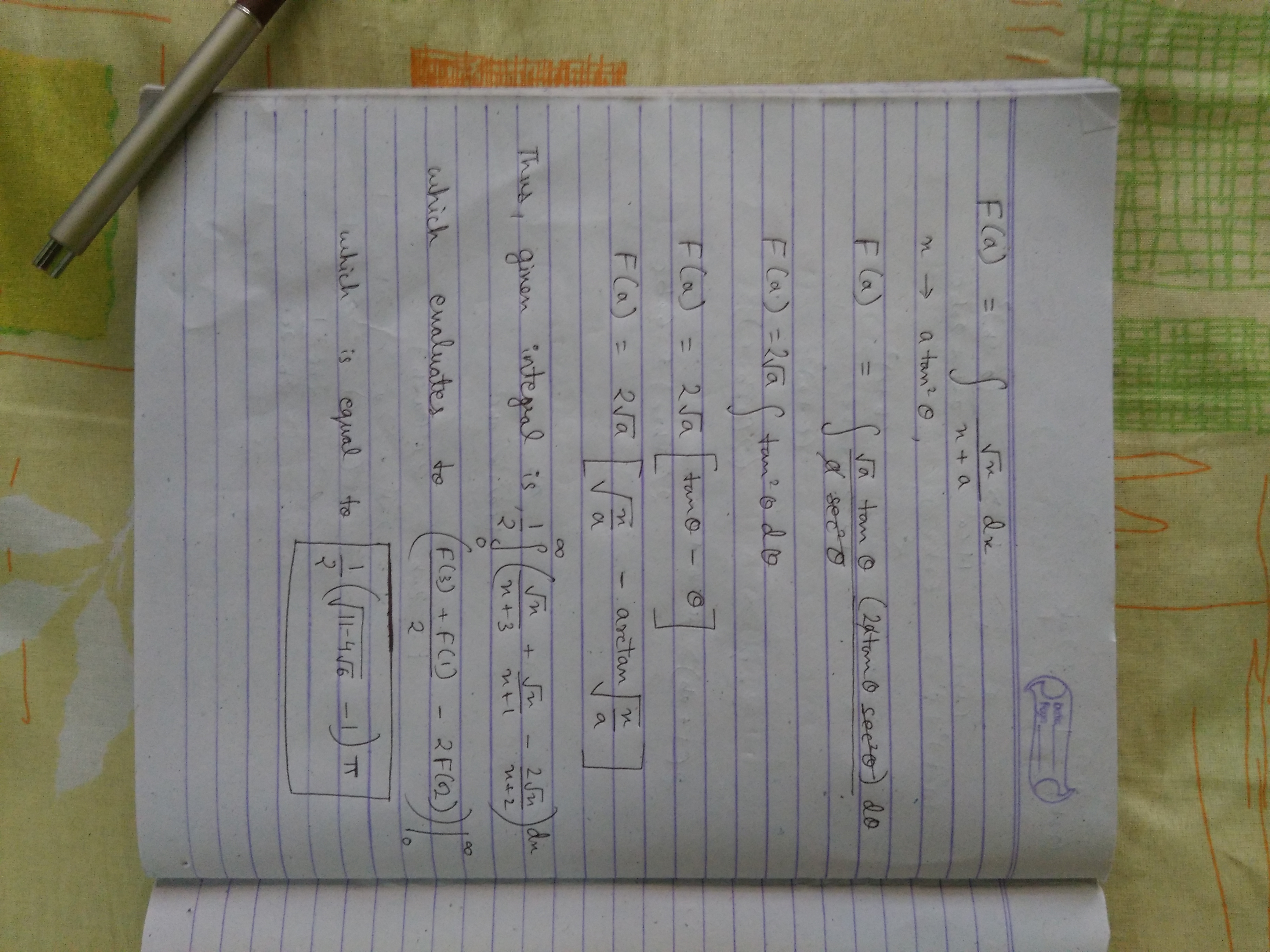- 5 years, 4 months ago

Problem 5:

Evaluate

$\displaystyle \int_{0}^{1} \dfrac{\ln (1+x)}{x}$

This problem was first solved by Aaryen Manzoor using a disallowed method and then solved by Aditya Kumar using a legal method. Credit is still given to Aaryen.

- 5 years, 4 months ago

solution to problem 5

replace x with -x to have $\int_0^{-1} \dfrac{\ln(1-x)}{x} dx$ $\sum_{n≥1} \dfrac{x^n}{n}=\ln(1-x)$ dividing by x and integrating from 0 to a $\int_0^{a} \dfrac{\ln(1-x)}{x} dx=\sum_{n≥1} \dfrac{a^n}{n^2}$ We have $\int_0^{-1} \dfrac{\ln(1-x)}{x} dx= \sum_{n≥1}\dfrac{(-1)^n}{n^2}=\sum_{n≥1} \dfrac{(-1)^n}{n^2}=\sum_{n≥1} \dfrac{1}{n^2}-2\sum_{n≥1} \dfrac{1}{(2n)^2}=\\\dfrac{1}{2}\sum_{n≥1} \dfrac{1}{n^2}=\boxed{\dfrac{\pi^2}{12}}$

- 5 years, 4 months ago

$\text{Alternate solution to Problem 5:}$Using MacLaurin expansion of $\ln(1+x)$:$\ln(1+x)=\sum_{r=1}^{\infty} (-1)^{r+1}\dfrac{x^r}{r}$$\Longrightarrow \dfrac{\ln(1+x)}{x}=\sum_{r=1}^{r=\infty}(-1)^{r+1}\dfrac{x^{r-1}}{r}$$\Longrightarrow I=\int_{0}^{1}(\sum_{r=1}^{r=\infty}(-1)^{r+1}\dfrac{x^{r-1}}{r})dx$$\Longrightarrow I=\sum_{r=1}^{\infty}(-1)^{r+1}\dfrac{\color{#D61F06}{1}}{r^2}=\dfrac{{\pi}^2}{12}$